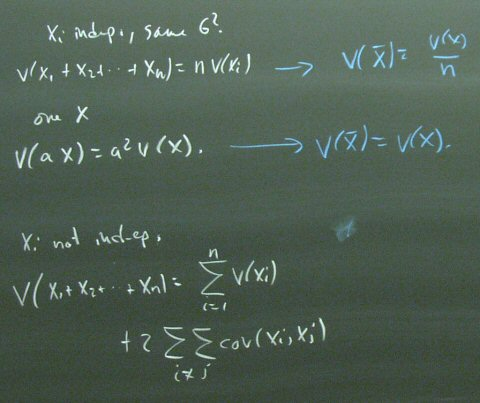## December 04, 2003

### Good Question

A student raised the issue of why we get different answers to 10 shares of stock X than for 1 share each of 10 stocks. This has to do with the rules for variances of sums. If random variables are independent, then the variance of a sum is the sum of the variances. If they are not independent, that rules does not apply. Ten shares of X are the exact opposite of independent. They are identical. The variance of 10 shares of X is 100 times the variance of one share of X.Posted by bparke at December 4, 2003 02:56 PM